# Differential Equations Flashcards

Differential Equations Flashcards
1/13 (missed) 0 0
Create Your Account To Continue Studying

As a member, you'll also get unlimited access to over 75,000 lessons in math, English, science, history, and more. Plus, get practice tests, quizzes, and personalized coaching to help you succeed.

Try it risk-free for 30 days. Cancel anytime.
###### Already registered? Login here for access
Solve the following: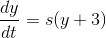The solution is: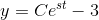Got it
Solve for the following for y: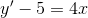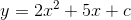Got it
Separation of Variables
Technique used to solve differential equations that involves isolating variables to their own sides of the equation. For example, only having x on one side of the equation and y on the other.
Got it
Solve the following for y: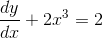The solution is: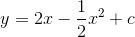Got it
Solve the following: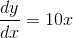The solution is: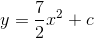Got it
Suppose the population of daisies in a field increases at the rate: ds/dt=1.5s (where s is the daisy population). Using this differential equation, find the population growth equation for daisies.
s = e1.5t
Got it

### Ready to move on?

or choose a specific lesson: See all lessons in this chapter
13 cards in set

## Flashcard Content Overview

Use this differential equations flashcard set to help you brush up on your calculus skills. Differential equations can be a difficult topic to master, but this flashcard set breaks down the fundamentals of what's involved when solving these equations. Techniques like separation of variables and differential notation are discussed, as well as more difficult application problems involving related rates. Use this flashcard set to study for your next calculus test or to ensure mastery of differential equations.

To learn more about differential equations, check out these lessons:

Front
Back
Suppose the population of daisies in a field increases at the rate: ds/dt=1.5s (where s is the daisy population). Using this differential equation, find the population growth equation for daisies.
s = e1.5t
Solve the following:The solution is:Solve the following for y:The solution is:Separation of Variables
Technique used to solve differential equations that involves isolating variables to their own sides of the equation. For example, only having x on one side of the equation and y on the other.
Solve for the following for y:Solve the following:The solution is:A cylindrical pool drains at a constant rate of dV/dt = 600 cm3/sec. Solve for how fast the height of the pool will change (dh/dt) if the pool has a radius of 500 cm. (V = pi*r 2*h)
dh/dt = 0.00076 cm/sec
Related Rate Problems
These problems involve finding the rate of change of a quantity by relating that rate to other known rates. A common related rate problem is the draining tank problem.
Distance Formula
The distance formula is: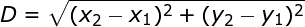The equation for a line is y = x3 + 3 and the x-coordinate is increasing at a rate of 1 unit/sec. When the point is (1,4), find the rate that the points distance from the origin is changing.
The rate (in units/sec) is: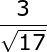Differential Equation
Differential equations relate a function to its derivative. In other words, it relates a function (and variables) to a rate of change.
Determine if the following is a differential equation.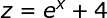No, this is not a differential equation. A differential equation must relate a variable to a rate of change.
Determine if the following is a differential equation.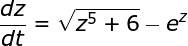Yes, this is a differential equation because it relates a variable and its rate of change.

To unlock this flashcard set you must be a Study.com Member.